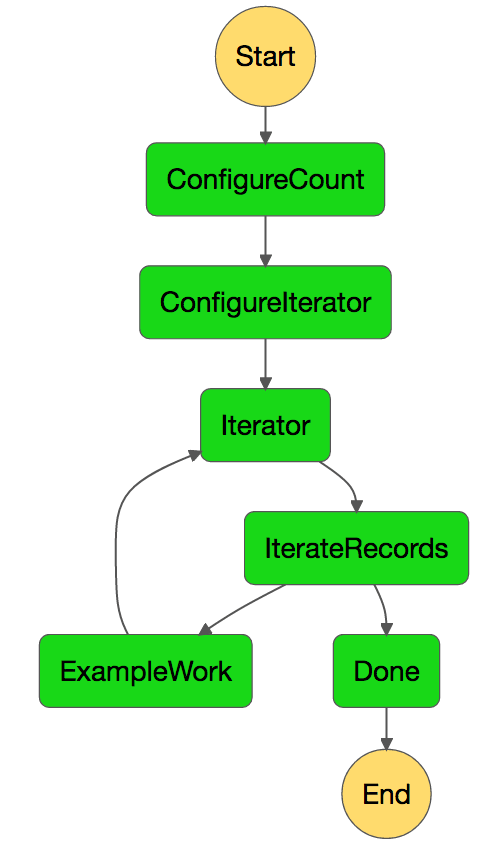## Iterating with AWS Step Functions

One interesting challenge I immediately encountered when attempting to work with AWS Lambda and Step functions was the need to process large files. Lambda functions have a couple of limitations namely memory and a 5 minute timeout. If you have some operation you need to perform on a very large dataset it may not be possible to complete this operation in a single execution of a lambda function. There are several ways to solve this problem, in this article I would like to demonstrate how to create an iterator pattern in an AWS Step Function as a way to loop over a large set of data and process it in smaller parts.In order to iterate we have created an Iterator Task which is a custom Lambda function. It accepts three values as inputs in order to operate: index, size and count.

Here is the code for this example step function:

[code language=”javascript”]
{
"Comment": "Iterator Example",
"StartAt": "ConfigureCount",
"States": {
"ConfigureCount": {
"Type": "Pass",
"Result": 10,
"ResultPath": "\$.count",
"Next": "ConfigureIterator"
},
"ConfigureIterator": {
"Type": "Pass",
"Result": {
"index": -1,
"step": 1
},
"ResultPath": "\$.iterator",
"Next": "Iterator"
},
"Iterator": {
"Resource": "arn:aws:lambda:{region}:{accountId}:function:iterator",
"ResultPath": "\$.iterator",
"Next": "IterateRecords"
},
"IterateRecords": {
"Type": "Choice",
"Choices": [
{
"Variable": "\$.iterator.continue",
"BooleanEquals": true,
"Next": "ExampleWork"
}
],
"Default": "Done"
},
"ExampleWork": {
"Type": "Pass",
"Result": {
"success": true
},
"ResultPath": "\$.result",
"Next": "Iterator"
},
"Done": {
"Type": "Pass",
"End": true
}
}
}
[/code]

### ConfigureCount

In this step we need to configure the number of times we want to iterate. In this case I have set the number of iterations to 10 and put it into a variable called \$.count. In a more complete example this may be the number of files you want to iterate over. For example in my real world scenario I am receiving a substantial CSV file which is then broken into many smaller CSV files, all stored in s3, the number of smaller files is then set into the count variable here. The large CSV file can be read entirely in a single lambda execution, streaming sections into smaller files, never loading the entire file into memory at the same time; but it cannot be processed entirely in a single function. Thus we split it and then iterate over the smaller parts.

### ConfigureIterator

Here we set the index and step variables into the \$.iterator field, which the iterator lambda uses to determine whether or not it should continue iterating.

### Iterator

This is the iterator itself, a small lambda function that simply increments the current index by the step size and calculates the continue field based on the current index and count.

[code language=”javascript”]
export function iterator (event, context, callback) {
let index = event.iterator.index
let step = event.iterator.step
let count = event.count

index += step

callback(null, {
index,
step,
count,
continue: index < count
})
}
[/code]

The reason why we want to support a step size is because we may have multiple workers which operate on data in parallel. In this example we have a single worker but in other cases we may need more in order to complete the overall work in a timely fashion.

### IterateRecords

From there we need to immediately move into a Choice state. This state simply looks at the \$.iterator.continue field and if it is not true then our iteration is over and we exit the loop. If iteration is not over then we move to the worker tasks which may use the \$.iterator.index field to determine which unit of work it should operate on.

### ExampleWork

In this example this is just a Pass state, but in a real example this may represent a series of Tasks or Activities which process the data for this iteration. When completed, the last step in the series should point back to the Iterator state.

Its also important to note that all states in this chain must use the ResultPath field to bucket their results in order to preserve the state of the iterator field throughout theses states. Do not override the \$.iterator or \$.count fields while doing work or you may end up in an infinite loop or error condition.

### Done

This state simply signifies the end of the step function.